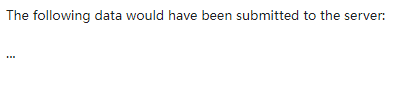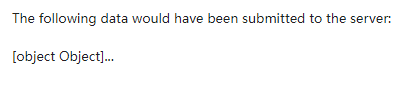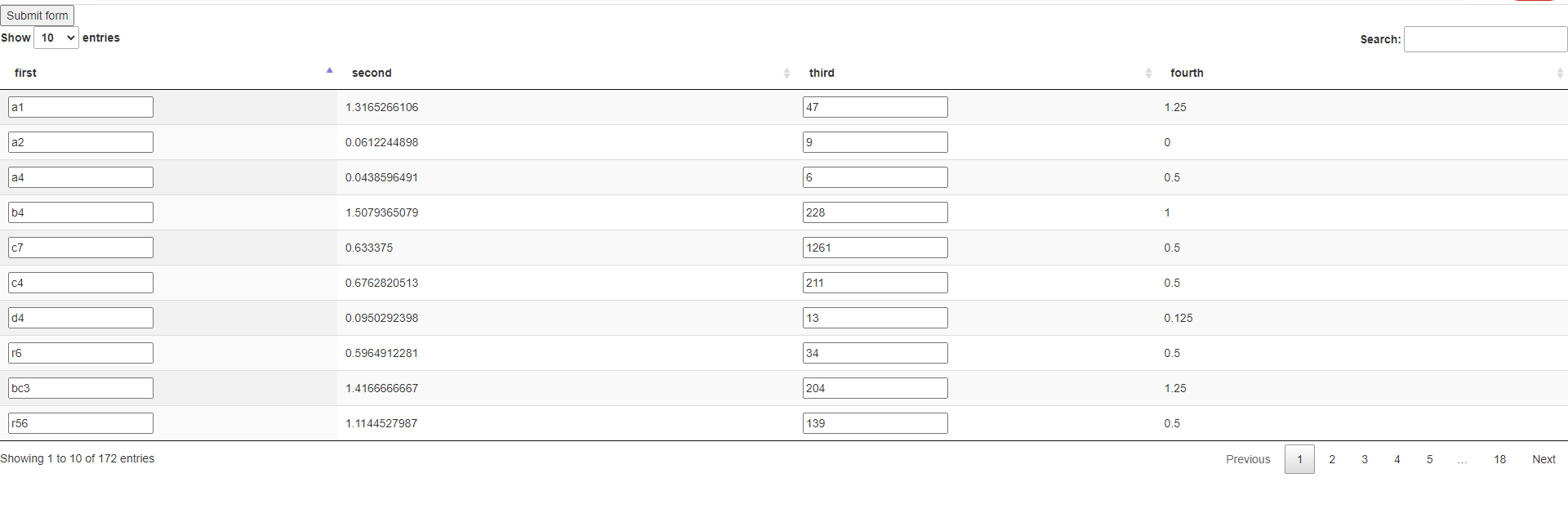# how can i get the form(input) data in datatable?

** code**:

``````    <script>
var table = \$('#example').DataTable({
"ajax": {
dataSrc: ""
},
"columns": [
{title:'first', data: 'first'},
{title:'second', data: 'second'},
{title:'third', data: 'third'},
{title:'fourth', data: 'fourth'}],
"columnDefs": [
{
targets: [0, 2],
render: function (data, type, row, meta) {
if (type === 'display') {
return '<input type="text" value="' + data + '">';
}
return data;
},
}
],
destroy: true
})
;

\$('button').click(function () {
var data = table.\$('input, select').serialize();
alert("The following data would have been submitted to the server: \n\n"+
data +'...'
);
});
});
</script>

<button type="submit">Submit form</button>
<table id="example" class="display" style="width:100%">
</table>
``````

Description of problem:

when i submit, it returnsseems that the data is empty...
how can i fix it?

• You are creating a text input with this:

``````return '<input type="text" value="' + data + '">';
``````

But you are trying to retrieve select inputs with this:

``````var data = table.\$('input, select').serialize();
``````

Try changing to this:

``````var data = table.\$('input, text').serialize();
``````

Kevin

• @kthorngren remain the same. data is empty.
is there something to do with how i create the text input or how i post data into datatable?
because when i change

var data = table.\$('input, text').serialize();

into

var data = table.data()

it returns sth below after submit•this is the DT